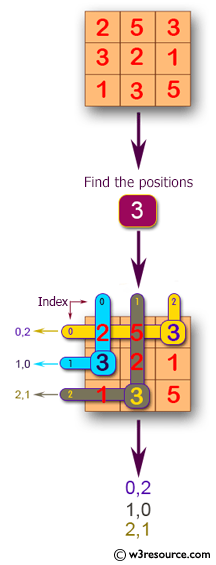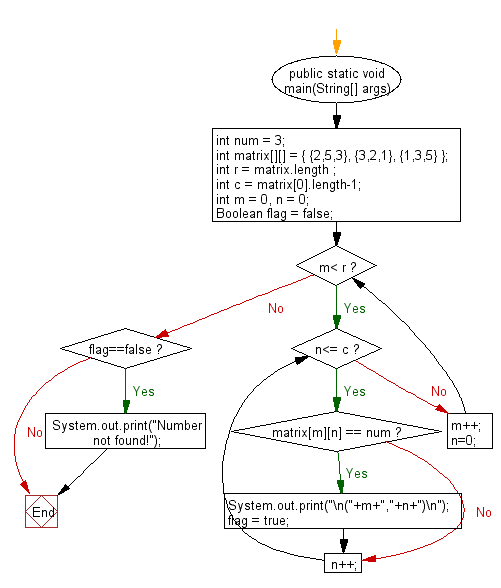﻿ Java programming exercises: Find the all positions of a given number in a given matrix - w3resource# Java Exercises: Find the all positions of a given number in a given matrix

## Java Basic: Exercise-194 with Solution

Write a Java program to find the all positions of a given number in a given matrix. If the number not found print ("Number not found!").

Pictorial Presentation:Sample Solution:

Java Code:

``````public class Solution {
public static void main(String[] args) {
int num = 3;
int matrix[][] = {
{2,5,3},
{3,2,1},
{1,3,5}
};
int r = matrix.length;
int c = matrix.length - 1;
int m = 0, n = 0;
Boolean flag = false;
while (m < r) {
while (n <= c) {
if (matrix[m][n] == num) {
System.out.print("\n(" + m + "," + n + ")\n");
flag = true;
}
n++;
}
m++;
n = 0;
}
if (flag == false)
}
}
```
```

Sample Output:

```(0,2)

(1,0)

(2,1)
```

Flowchart:Java Code Editor:

Company:

What is the difficulty level of this exercise?

﻿

## Java: Tips of the Day

Parsing dates:

```import java.io.*;
import java.util.*;
import java.text.*;

String s = "2001/09/23 14:39";

SimpleDateFormat formatter = new SimpleDateFormat ("yyyy/MM/dd H:mm");
Date d = formatter.parse(s, new ParsePosition(0));
```